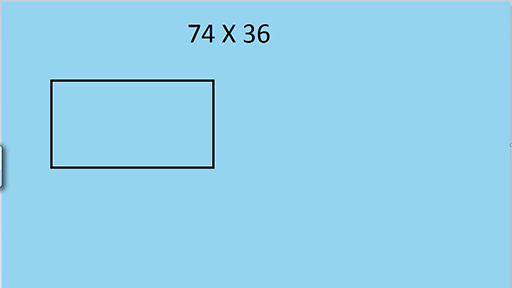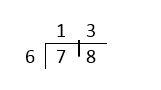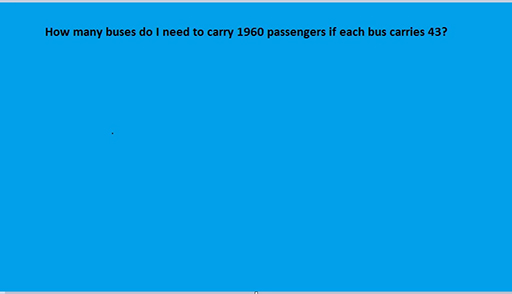Education & Development

### Become an OU studentTeaching mathematics

Start this free course now. Just create an account and sign in. Enrol and complete the course for a free statement of participation or digital badge if available.

# 3.3 Multiplication and division

The next video demonstrates two methods for long multiplication and discusses the pros and cons of each method.

Download this video clip.Video player: Video 5 Two methods for long multiplicationVideo 5 Two methods for long multiplication
Interactive feature not available in single page view (see it in standard view).

## Division

Division is the arithmetic operation where learners experience the most difficulty. Division is the inverse of multiplication which means that it is possible to approach division by complementary multiplying. For example, to answer the question 78 ÷ 6 you can ask ‘How many 6s make 78?’.

One way to answer the question in this way is to use the ‘chunking’ method where you add ‘chunks’ of 6 until you reach 78. It saves having to add 6 at a time, which could be laborious. For example:

(you know that 78 is more than 6 lots of 10, so start there)

(you have 18 left to make the 60 up to 78, that’s 3 lots of 6)

So

Or you could start with 78 and keep subtracting 6 until you have nothing (or a number smaller than 6) left: For example:

78

78 ÷ 6 calculating by subtracting sixes

Whether working up to 78 or down from 78, the method is basically finding how many 6s there are in 78. This method clearly becomes harder with larger numbers and requires knowledge of multiplication tables and good mental arithmetic. It is, however, an intuitive method of dividing.

The traditional method of division is known among teachers as either the bus stop method (because the working looks like a bus stop),or the ‘gazinta’ method (because you ask how many times 6 ‘goes into’ each number).Figure 5 One formal method of short division

Figure 5 shows that 6 goes into 7 once with 1 left over. So, put the 1 (for the once) above the 7 and the 1 left over next to the 8. Now read it as 6 goes into 18, which is 3. Put the 3 over the 8. This is an easy method to operate if you can remember the procedure. It is not so easy to understand why it works. Yet many learners prefer it to having to use the ‘chunking’ method.

The bus stop method is efficient to use with larger numbers as well, as long as your multiplication tables are good, and you can do some mental calculation. When dividing by two-digit numbers, I prefer to use and to teach a version of this short division method.

## Activity 9 Solving a division problem

Timing: Allow 10 minutes

Suppose you have 1960 pupils and teachers in a school to ferry to an event in buses which each carry 43 passengers. How many buses will be needed?

So, you are trying to find out how many times 43 goes into 1960 and you need an extra bus for the remainders.

Spend ten minutes ‘having a go’, using whichever method you prefer. Then watch the video.

Download this video clip.Video player: Video 6 Two ways to solve a division problemVideo 6 Two ways to solve a division problem
Interactive feature not available in single page view (see it in standard view).